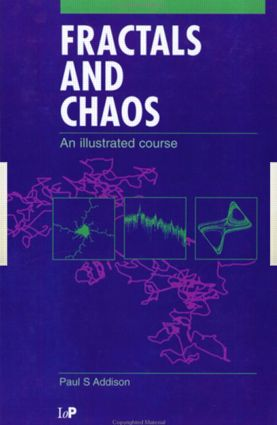Fractals and Chaos

An illustrated course, 1st Edition

CRC Press

256 pages

Purchasing Options:\$ = USD
Paperback: 9780750304009
pub: 1997-01-01
SAVE ~\$16.79
\$83.95
\$67.16
x
Hardback: 9781138417618
pub: 2018-06-28
SAVE ~\$39.00
\$195.00
\$156.00
x

FREE Standard Shipping!

Description

Fractals and Chaos: An Illustrated Course provides you with a practical, elementary introduction to fractal geometry and chaotic dynamics-subjects that have attracted immense interest throughout the scientific and engineering disciplines. The book may be used in part or as a whole to form an introductory course in either or both subject areas. A prominent feature of the book is the use of many illustrations to convey the concepts required for comprehension of the subject. In addition, plenty of problems are provided to test understanding. Advanced mathematics is avoided in order to provide a concise treatment and speed the reader through the subject areas. The book can be used as a text for undergraduate courses or for self-study.

Reviews

"Fractals and Chaos: An Illustrated Course is well designed for self-study, making it a great practical resource for those working in the physical sciences or engineering as well as for students."

—Danny Yee’s Book Reviews, February 2016

INTRODUCTION

Introduction

A matter of fractals

Deterministic chaos

Chapter summary and further reading

REGULAR FRACTALS AND SELF-SIMILARITY

Introduction

The Cantor set

Non-fractal dimensions: the Euclidean and topological dimension

The similarity dimension

The Koch curve

The quadratic Koch curve

The Koch island

Curves in the plane with similarity dimension exceeding 2

The Sierpinski gasket and carpet

The Menger Sponge

Chapter summary and further reading

Revision questions and further tasks

RANDOM FRACTALS

Introduction

Randomizing the Cantor set and Koch curve

Fractal boundaries

The box counting dimension and the Hausdorff dimension

The structured walk technique and the divider dimension

The perimeter-area relationship

Chapter summary and further reading

Revision questions and further tasks

REGULAR AND FRACTIONAL BROWNIAN MOTION

Introduction

Regular Brownian motion

Fractional Brownian motion: time traces

Fractional Brownian surfaces

Fractional Brownian motion: spatial trajectories

Diffusion limited aggregation

The color and power and noise

Chapter summary and further reading

Revision questions and further tasks

ITERATIVE FEEDBACK PROCESSES AND CHAOS

Introduction

Population growth and the Verhulst model

The logistic map

The effect of variation in the control parameter

General form of the iterated solutions of the logistic map

Graphical iteration of the logistic map

Bifurcation, stability and the Feigenbaum number

A two dimensional map: the Henon model

Iterations in the complex plane: Julia sets and the Mandelbrot set

Chapter summary and further reading

Revision questions and further tasks

CHAOTIC OSCILLATIONS

Introduction

A simple nonlinear mechanical oscillator: the Duffing oscillator

Chaos in the weather: the Lorenz model

The Rossler systems

Phase space, dimension and attractor form

Spatially extended systems: coupled oscillators

Spatially extended systems: fluids

Mathematical routes to chaos and turbulence

Chapter summary and further reading

Revision questions and further tasks

CHARACTERIZING CHAOS

Introduction

Preliminary characterization: visual inspection

Preliminary characterization: frequency spectra

Characterizing chaos: Lyapunov exponents

Characterizing chaos: dimension estimates

Attractor reconstruction

The embedding dimension for attractor reconstruction

The effect of noise

Regions of behavior on the attractor and characterization limitations

Chapter summary and further reading

Revision questions and further task

APPENDIX 1: Computer Program for Lorenz Equations

APPENDIX 2: Illustrative Papers

APPENDIX 3: Experimental Chaos

SOLUTIONS

REFERENCES